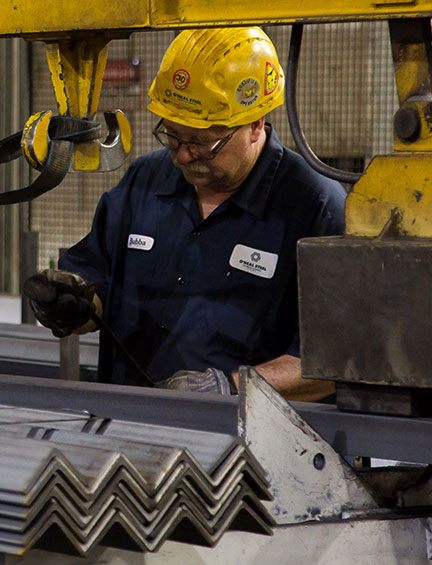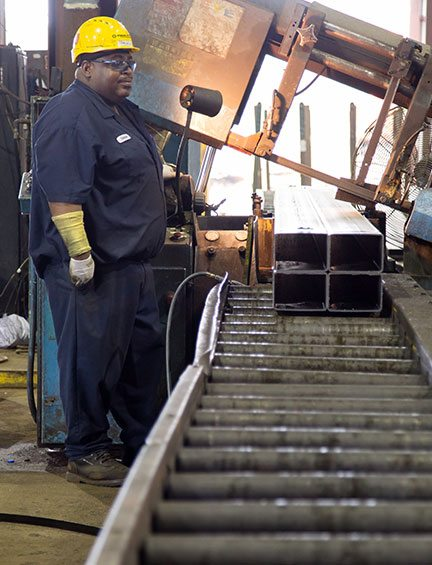##### Calculated Weights

*These weights should be used for estimation purposes only. Plate weight does not allow for kerf.

Inches to Millimeter
 Inches = Millimeter =
Millimeter to Inches
 Millimeter = Inches =
Pounds to Kilograms
 Pounds = Kilograms =
Kilograms to Pounds
 Kilograms = Pounds =
Metric Tons to Pounds
 Metric Tons = Pounds =
Metric Tons to Tons
 Metric Tons = Tons =
Fahrenheit to Celsius
 Fahrenheit = Celsius =
Celsius to Fahrenheit
 Celsius = Fahrenheit =
Ff/Lbs to Joules
 ft/lbs = Joules =
Joules to Ft/lbs
 Joules = ft/lbs =
MPa to psi
 MPa = psi =
MPa to ksi
 MPa = ksi =
psi to MPa
 psi = MPa =
ksi to MPa
 ksi = MPa =
##### Carbon Equivalency Formula
 C = V = Mn = Cu = Cr = Ni = Mo = Carbon Equiv =

##### PcM Formula
 C = Ni = Si = Mo = Mn = V = Cu = B = Cr = PcM =01 / Browse by Type

### The Metal You Need

We carry a wide range of metal types for all manner of industries, including aluminum, stainless steel, hot rolled, cold-finished, and alloy.02 / Browse by Shape

### When You Need It

We carry a broad range of metal products in all shapes and sizes, meaning you can always find what you need to get the job done.03 / Processing Services

### How You Need It

No matter what products you need, whether custom in size or shape, O’Neal Steel’s processing services can work with your specifications.